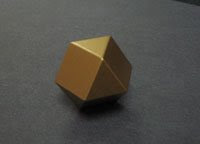## Tuesday, April 14, 2009

### 14

14 = 2 x 7.

14 is the sum of the 2nd, 3rd, 4th, and 5th numbers: 2 + 3 + 4 + 5 = 14.

14 is the smallest number n such that n and 2n end with the same digit: 214 = 16,384.

14 is the largest number of regions into which four circles can divide a plane.The cuboctahedron is an Archimedean solid with
14 faces (eight triangles and six squares).Source:
Wikipedia

#### 1 comment:

Ed Sandifer said...

Is there a typo here" It says "14 is the smallest number n such that n and 2n end with the same digit: 2^14 = 16,384." Shouldn't it also say "... smallest number n such that n and 2^n end ..."